# 5TH ANNIVERSARY AUCTION

### Auction Details

Choose Your Layout >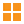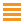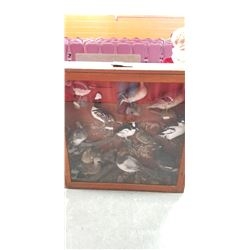202 - Shelf One
CDC Auctions
Shelf One
Bidding Has Concluded

Sold to f***r for (190.00 + 24.70) x 1 = 214.70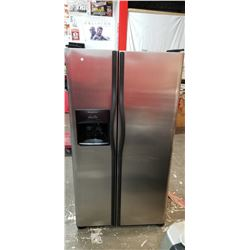203 - Shelf One
CDC Auctions
Shelf One
Bidding Has Concluded

Sold to f***r for (120.00 + 15.60) x 1 = 135.60204 - Shelf One
CDC Auctions
Shelf One
Bidding Has Concluded

Sold to f***r for (100.00 + 13.00) x 1 = 113.00205 - Shelf One
CDC Auctions
Shelf One
Bidding Has Concluded

Sold to f***r for (90.00 + 11.70) x 1 = 101.70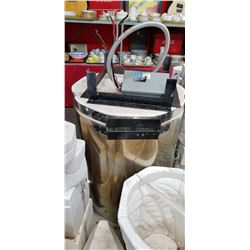206 - Shelf One
CDC Auctions
Shelf One
Bidding Has Concluded

Sold to f***r for (55.00 + 7.15) x 1 = 62.15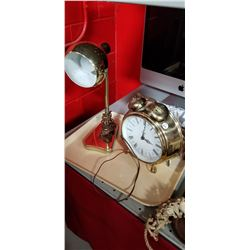207 - Shelf One
CDC Auctions
Shelf One
Bidding Has Concluded

Sold to f***r for (20.00 + 2.60) x 1 = 22.60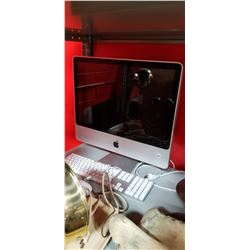208 - Shelf One
CDC Auctions
Shelf One
Bidding Has Concluded

Sold to f***r for (350.00 + 45.50) x 1 = 395.50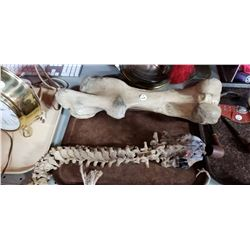209 - Shelf One
CDC Auctions
Shelf One
Bidding Has Concluded

Sold to f***r for (27.50 + 3.58) x 1 = 31.08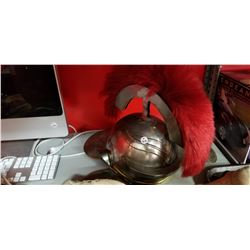210 - Shelf One
CDC Auctions
Shelf One
Bidding Has Concluded

Sold to f***r for (45.00 + 5.85) x 1 = 50.85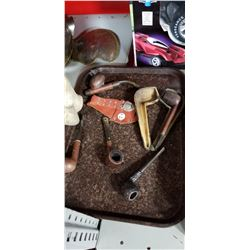211 - Shelf One
CDC Auctions
Shelf One
Bidding Has Concluded

Sold to f***r for (35.00 + 4.55) x 1 = 39.55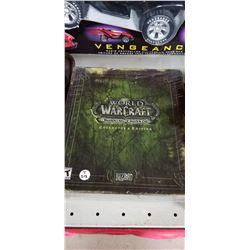212 - Shelf One
CDC Auctions
Shelf One
Bidding Has Concluded

Sold to f***r for (80.00 + 10.40) x 1 = 90.40213 - Shelf One
CDC Auctions
Shelf One
Bidding Has Concluded

Sold to f***r for (40.00 + 5.20) x 1 = 45.20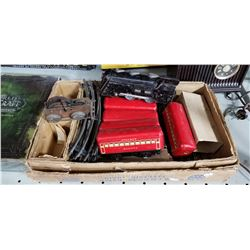214 - Shelf One
CDC Auctions
Shelf One
Bidding Has Concluded

Sold to f***r for (50.00 + 6.50) x 1 = 56.50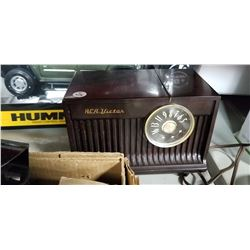215 - Shelf Two
CDC Auctions
Shelf Two
Bidding Has Concluded

Sold to f***r for (22.50 + 2.93) x 1 = 25.43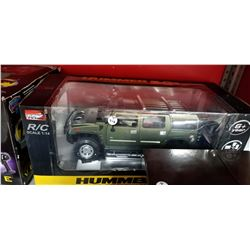216 - Shelf One
CDC Auctions
Shelf One
Bidding Has Concluded

Sold to f***r for (40.00 + 5.20) x 1 = 45.20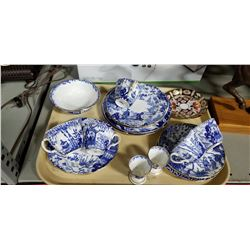217 - Shelf One
CDC Auctions
Shelf One
Bidding Has Concluded

Sold to f***r for (60.00 + 7.80) x 1 = 67.80218 - Shelf One
CDC Auctions
Shelf One
Bidding Has Concluded

Sold to f***r for (140.00 + 18.20) x 1 = 158.20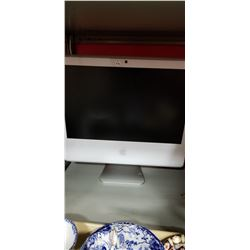219 - Shelf One
CDC Auctions
Shelf One
Bidding Has Concluded

Sold to f***r for (65.00 + 8.45) x 1 = 73.45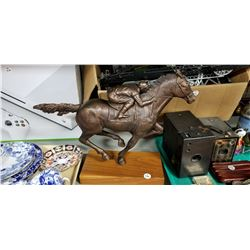220 - Shelf One
CDC Auctions
Shelf One
Bidding Has Concluded

Sold to f***r for (27.50 + 3.58) x 1 = 31.08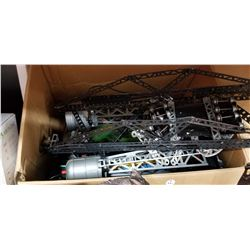221 - Shelf One
CDC Auctions
Shelf One
Bidding Has Concluded

Sold to f***r for (32.50 + 4.23) x 1 = 36.73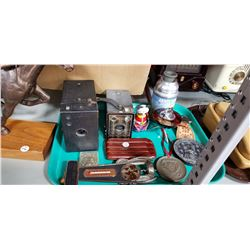222 - Shelf One
CDC Auctions
Shelf One
Bidding Has Concluded

Sold to f***r for (32.50 + 4.23) x 1 = 36.73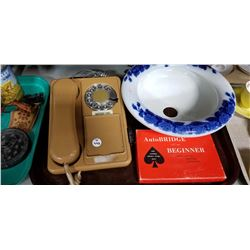223 - Shelf One
CDC Auctions
Shelf One
Bidding Has Concluded

Sold to f***r for (10.00 + 1.30) x 1 = 11.30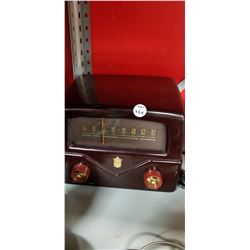224 - Shelf One
CDC Auctions
Shelf One
Bidding Has Concluded

Sold to j*****R for (45.00 + 5.85) x 1 = 50.85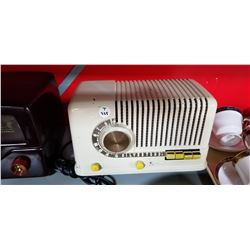225 - Shelf One
CDC Auctions
Shelf One
Bidding Has Concluded

Sold to f***r for (37.50 + 4.88) x 1 = 42.38226 - Shelf One
CDC Auctions
Shelf One
Bidding Has Concluded

Sold to f***r for (17.50 + 2.28) x 1 = 19.78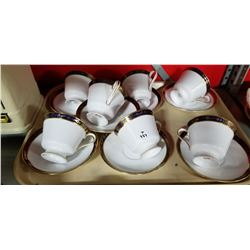227 - Shelf One
CDC Auctions
Shelf One
Bidding Has Concluded

Sold to f***r for (5.00 + 0.65) x 1 = 5.65228 - Shelf One
CDC Auctions
Shelf One
Bidding Has Concluded

Sold to f***r for (32.50 + 4.23) x 1 = 36.73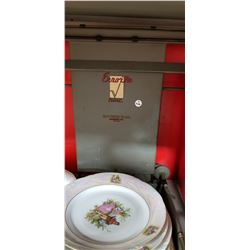229 - Shelf One
CDC Auctions
Shelf One
Bidding Has Concluded

Sold to f***r for (5.00 + 0.65) x 1 = 5.65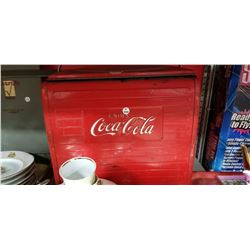230 - Shelf One
CDC Auctions
Shelf One
Bidding Has Concluded

Sold to f***r for (60.00 + 7.80) x 1 = 67.80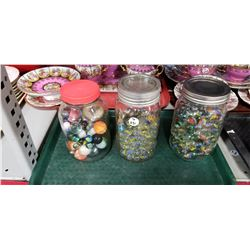231 - Shelf One
CDC Auctions
Shelf One
Bidding Has Concluded

Sold to f***r for (10.00 + 1.30) x 1 = 11.30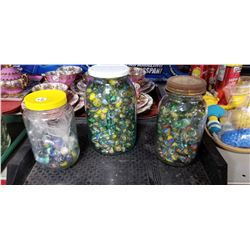232 - Shelf One
CDC Auctions
Shelf One
Bidding Has Concluded

Sold to f***r for (7.50 + 0.98) x 1 = 8.48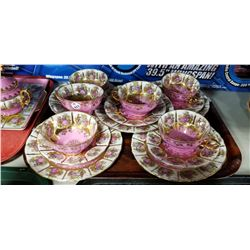233 - Shelf Two
CDC Auctions
Shelf Two
Bidding Has Concluded

Sold to f***r for (260.00 + 33.80) x 1 = 293.80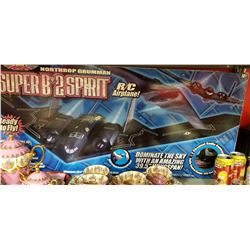234 - Shelf One
CDC Auctions
Shelf One
Bidding Has Concluded

Sold to f***r for (45.00 + 5.85) x 1 = 50.85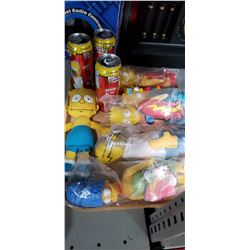235 - Shelf One
CDC Auctions
Shelf One
Bidding Has Concluded

Sold to s********F for (10.00 + 1.30) x 1 = 11.30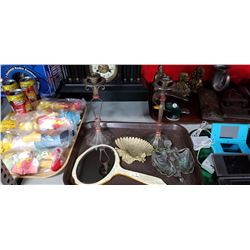236 - Shelf One
CDC Auctions
Shelf One
Bidding Has Concluded

Sold to f***r for (27.50 + 3.58) x 1 = 31.08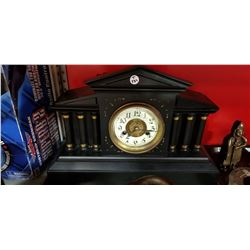237 - Shelf One
CDC Auctions
Shelf One
Bidding Has Concluded

Sold to f***r for (40.00 + 5.20) x 1 = 45.20238 - Shelf One
CDC Auctions
Shelf One
Bidding Has Concluded

Sold to f***r for (17.50 + 2.28) x 1 = 19.78239 - Shelf One
CDC Auctions
Shelf One
Bidding Has Concluded

Sold to f***r for (27.50 + 3.58) x 1 = 31.08240 - Shelf One
CDC Auctions
Shelf One
Bidding Has Concluded

Sold to f***r for (27.50 + 3.58) x 1 = 31.08241 - Shelf One
CDC Auctions
Shelf One
Bidding Has Concluded

Sold to f***r for (40.00 + 5.20) x 1 = 45.20242 - Shelf One
CDC Auctions
Shelf One
Bidding Has Concluded

Sold to f***r for (17.50 + 2.28) x 1 = 19.78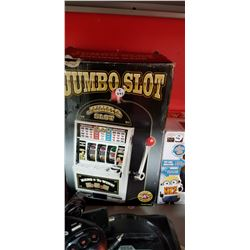243 - Shelf One
CDC Auctions
Shelf One
Bidding Has Concluded

Sold to f***r for (15.00 + 1.95) x 1 = 16.95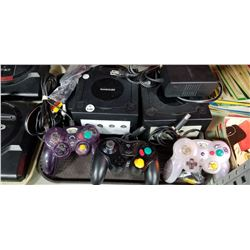244 - Shelf One
CDC Auctions
Shelf One
Bidding Has Concluded

Sold to f***r for (40.00 + 5.20) x 1 = 45.20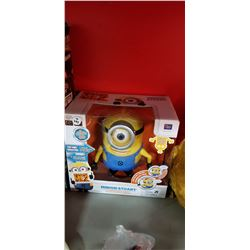245 - Shelf One
CDC Auctions
Shelf One
Bidding Has Concluded

Sold to j*****R for (17.50 + 2.28) x 1 = 19.78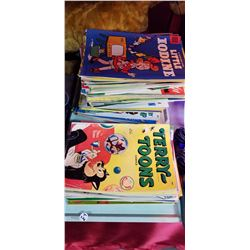246 - Shelf One
CDC Auctions
Shelf One
Bidding Has Concluded

Sold to f***r for (75.00 + 9.75) x 1 = 84.75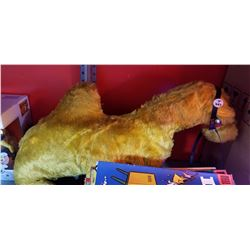247 - Shelf One
CDC Auctions
Shelf One
Bidding Has Concluded

Sold to f***r for (12.50 + 1.63) x 1 = 14.13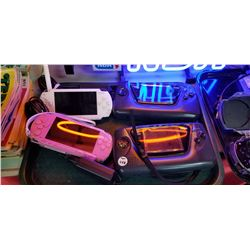248 - Shelf One
CDC Auctions
Shelf One
Bidding Has Concluded

Sold to f***r for (37.50 + 4.88) x 1 = 42.38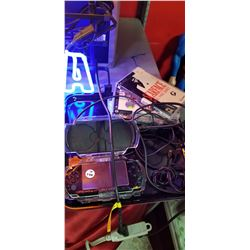249 - Shelf One
CDC Auctions
Shelf One
Bidding Has Concluded

Sold to f***r for (60.00 + 7.80) x 1 = 67.80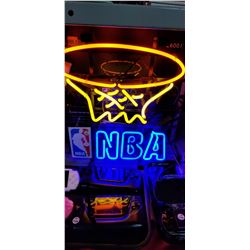250 - Shelf One
CDC Auctions
Shelf One
Bidding Has Concluded

Sold to f***r for (95.00 + 12.35) x 1 = 107.35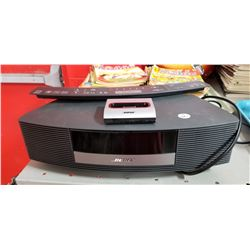251 - Shelf Two
CDC Auctions
Shelf Two
Bidding Has Concluded

Sold to f***r for (110.00 + 14.30) x 1 = 124.30